# How to Find the Equation of a Circle

Lesson Transcript
Instructor: Lance Cain
There are two commonly used forms of equations for a circle, in this lesson we'll take a few minutes to examine and understand both forms, and be able to convert from one to the other. Updated: 11/13/2019

## Circles

If you swing a ball attached to the end of a rope over your head like a cowboy's lasso, the ball travels in a circle with your hand at the center and the length of the rope as the radius, which you can see in the figure below.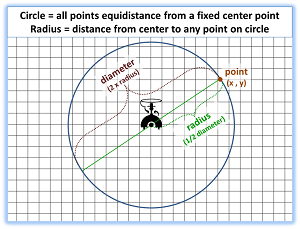We can use the location of your hand (the center) and the length of the rope (radius) to write an equation for the circular path of the ball. Here's how.

When we trace the circle on a grid like in the figure that we just saw, with the origin (0,0) at the center, we can see that any point (x,y) on the circle can be defined by drawing a right triangle and using the Pythagorean Theorem, which, remember, is (a^2 + b^2 = c^2).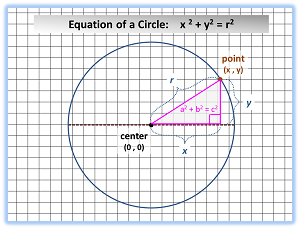The side 'a' is the horizontal distance (x); side 'b' is the vertical distance (y); and the hypotenuse of the triangle is the radius of the circle (r). Substituting these distances from the circle into the Pythagorean Theorem, we obtain the standard form of the equation for a circle:

x^2 + y^2 = r^2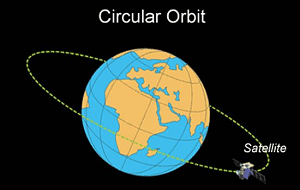However, what if the circle is not centered on the origin? What if the center of the circle is at point (9,5) instead of (0,0)? No sweat, a small adjustment to our equation will allow for the center to be anywhere. Simply subtract the x-value and y-value of the center point from the x and y variables in our equation. For example, to find points on a circle centered at (9,5), we would re-write the equation as:

(x-9)^2 + (y-5)^2 = r^2

An error occurred trying to load this video.

Try refreshing the page, or contact customer support.

Coming up next: Radian Measure: Definition & Formula

### You're on a roll. Keep up the good work!

Replay
Your next lesson will play in 10 seconds
• 0:04 Circles
• 1:45 Standard Form Vs General Form
• 2:37 Some Examples
• 8:08 Lesson Summary
Save Save

Want to watch this again later?

Log in or sign up to add this lesson to a Custom Course.

Timeline
Autoplay
Autoplay
Speed Speed

## Standard Form vs. General Form

If we assume a generic center point like (s,t), we write our equation in what is known as the standard form equation for a circle, which, as you can see, is (x - s)^2 + (y - t)^2 = r^2. With the use of a little algebra to expand and combine like terms, we can convert the standard form into the following, known as the general form of the equation for a circle, which, as you can see, is: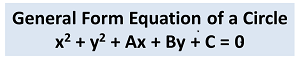In the general form of the equation, the coefficients of the x and y terms - A and B, respectively - can be any real numbers. Both, the standard and general forms of the equation produce a circle when graphed; the information you have been given will determine which equation is best to use.

## Some Examples

Here is Example 1:

Write the standard form of the equation for a circle centered at point (3 , 7) and a radius of 10.

Solution:

You need to insert x and y values and set equal to the radius squared:

(x - 3)^2 + (y - 7)^2 = 10^2 ===> (x - 3)^2 + (y - 7)^2 = 100

And convert the standard form equation to general form as follows.

First we expand:

(x - 3)^2 + (y - 7)^2 = 100 ===> x^2 - 6x + 9 + y^2 -14y + 49 = 100

Then we rearrange terms:

x^2 + y^2 - 6x -14y + 9 +49 = 100 ===> x^2 + y^2 - 6x -14y + 58 = 100

And then we set equal to zero:

x^2 + y^2 - 6x -14y + 58 - 100 = 100 - 100 ===> x^2 + y^2 - 6x -14y - 42 = 0

Here is Example 2:

What is the radius and center point of the circle in the figure below? We first write the equation for the circle in general form.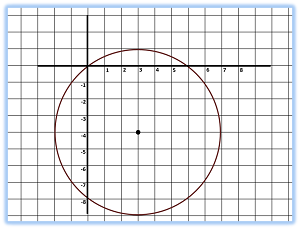Solution:

radius = 5

center point = (3 , -4)

To write the equation for this circle in general form, we first write down the standard form of the equation, which is:

(x - 3)^2 + (y + 4)^2 = 25

Expand the equation and combine like terms.

Expand:

(x - 3)^2 and (y + 4)^2

x^2 - 6x +9 +y^2 + 8y + 16= 25

Now you arrange terms:

x^2 + y^2 - 6x + 8y + 25 = 25

And set equal to zero:

x^2 + y^2 - 6x + 8y + 25 - 25 = 25 - 25 ===> x^2 + y^2 - 6x + 8y = 0

Now here's Example 3:

To unlock this lesson you must be a Study.com Member.
Create your account

### Register to view this lesson

Are you a student or a teacher?

### Unlock Your Education

#### See for yourself why 30 million people use Study.com

##### Become a Study.com member and start learning now.
Back
What teachers are saying about Study.com
Create an account to start this course today
Used by over 30 million students worldwide